We now know that a car generates aerodynamic forces that depend on the square of its speed with respect to the air. The magnitude of these forces is not only linked to car-to-air velocity, but also on car’s dimensions, shape and on specific features.

Although each small portion of a car contributes to defining the aerodynamic forces applied by air to it, some components have a more dominant role in this respect. The following picture, referring to 2009 spec Formula 1 and taken from “formula1-dictionary.net”, gives us an idea about the portion of the overall drag and downforce that can be linked to the main aerodynamic components of a car. As we see, the most important components in terms of aerodynamics are the two wings, the wheels, the bodywork and the diffuser.

Starting with the wheels, we immediately recognize that they not only create lift (or negative downforce, a force pushing upward and something we would really not like to have in a race car), but they are also responsible for a very important portion of a Formula 1 drag. The situation is slightly better for an LMP or GT vehicle since the wheels are partially hidden by the bodywork and are not directly exposed to the airflow.

Besides this, the picture suggests how the main contributors to downforce and drag are actually the wings and the diffusers. In the following part of this article, we will try to understand how a generic wing and diffuser work.

The theory relative to wings can be traced back, again, to their role and importance in the aeronautic industry. A racecar wing works principally the same way as an aeroplane one, with the only difference being that in a racecar wings lie normally rotated by 180 degrees in order to generate downforce, not lift.

The following picture (from “www.allstar.fiu.edu”) shows a generic wing profile and identifies its main elements.

As can be seen, a wing has an upper and a lower surface (no matter in which direction the wing is turned!) and they are both curved. Their curvature can be the same, or they can have a non-symmetric section (as in the picture), with different curvatures on the two sides. The nose of the wing, where air meets its profile first, is called leading edge and it normally has a rounded shape. The ending point of the wing is called trailing edge and has normally a kinked shape.

The chord is the straight line connecting leading and trailing edge. If the midline of the wing is not straight (it doesn’t coincide with wing’s chord), we say the wing has a camber. All non-symmetric wings fall in this group.

There is some misconception about how wings work. Sometimes, the way they generate forces is explained using Bernoulli’s theorem, a very important theorem for fluid dynamics and aerodynamics. This theorem is summarized by the following equation: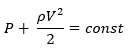Where:

• P is fluid pressure;
• ρ is fluid density;
• V is fluid velocity.

The equation above basically tells us that, if an incompressible fluid (as air is, at least below sonic speed) increases its speed, its pressure must decrease (of course the opposite is also true: if its speed is lower, its pressure must be higher).

Who uses this theorem to explain how wings work, basically consider two particles of fluid approaching at the same time wings leading edge and then taking two different paths, one flowing around the wing top surface and one around the lower surface. It is then assumed that the two particles will leave the wing at the same time. Since one surface offers a longer path than the other, the portion of the fluid flowing around the longer side must do this faster than the portion flowing around the shorter side, so that they can “meet” again when they leave the wing (at the same time). Having a portion of the fluid at a higher speed than the other, because of Bernoulli’s theorem, it should have a lower pressure (the opposite is true for the other side). The difference of pressure would generate a force, lifting and breaking the wing.

Although this approach leads to the right conclusions, it is based on a wrong assumption:  Bernoulli’s theorem is only valid in a closed volume; assuming that the flow around a wing moves in a closed volume is fundamentally wrong, hence Bernoulli’s theorem cannot be applied to this physics problem.

In other words, it is not true that the two particles will meet again at the leading edge of the wing, leaving it at the same time. Why should they, after all? Experiments done on a simple wing, using very high framerate cameras and fluid visualization tools to follow and record what the airstream around a wing looks like, demonstrate that this is indeed not true.We can get a feeling about why this explanation is wrong if we consider a very thin, curved body and we analyze how a fluid moves around it. If the thickness is very small, the path followed by air flowing on the two sides of our body will have approximately the same length (and, hence, the same speed), but this part will anyway generate aerodynamic forces. We had an example of something similar some years ago in LMP1, in the rear wind endplate area (see image below, courtesy of Mulsannescorner.com, focusing on element number 2):

As we mentioned in our previous article, a body generates aerodynamic forces because it is able to create a pressure differential in the air around it. A wing generates a pressure differential mainly because of air’s viscosity, not because of Bernoulli’s theorem.

To understand how a wing truly works, we have to remember that, according to physics basic laws, if a mass accelerates, there must be a force acting on it. We know that an acceleration exists any time a mass moves on a curved path, even if at constant tangent velocity.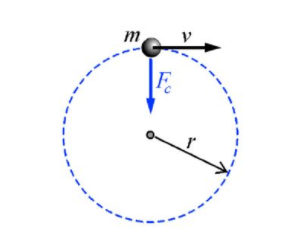In our image, Fc, the force accelerating the mass toward the centre of its curved path, is defined by the following equation:Where:

• Fc is the centripetal force;
• m is our mass;
• v is the tangential speed;
• r is the radius of mass circular path.

Analogously, we can conclude that if a fluid flows following a curved path, there must be a force acting on its elements and letting them accelerate toward the centre of the curved trajectory.

According to what we have described in our previous article, we know that, because this could happen, there must be a pressure difference producing a net Force.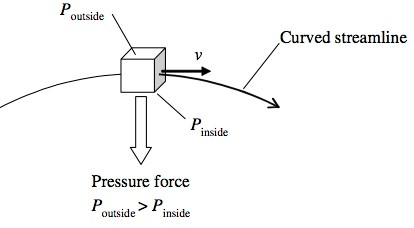This is exactly what a wing does to air when moving through it. Because of its shape and its angle of attack and because of air’s viscosity, a wing “bends” the flow, creating a pressure differential and, hence, a net force.

Air particles will follow a curved path, after meeting a wing, mainly because of air’s viscosity: some of the particles will adhere to wings upper and lower surfaces, creating a boundary layer.Because of the friction between air and wing, air will follow a curved path, because it will try to stick to wing’s surfaces (and this will indeed happen as long the angle of attack or air relative speed don’t exceed a certain value).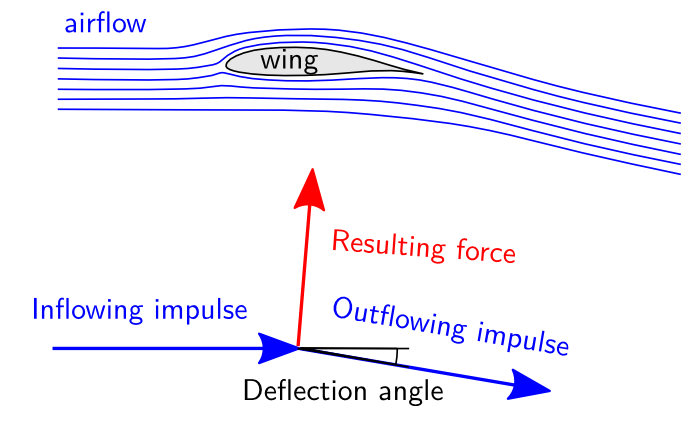Bernoulli’s principle is a better explanation of how race cars diffusers work. Although Bernoulli’s theorem doesn’t catch all that happens in a diffuser, it helps to understand one of its main working principles.

A diffuser is essentially a device, part of car’s underbody, composed mainly by a side-view inclined surface, which starts rising, along a car’s wheelbase, as far in front of rear wheel centre as rules allow.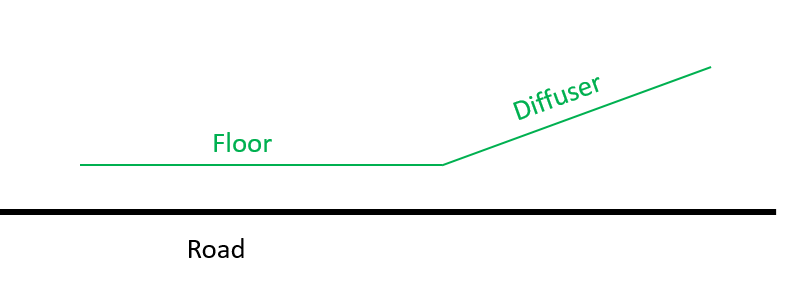The most important role of a diffuser is to accelerate the airflow below the car, thus generating a low-pressure area and, hence, downforce.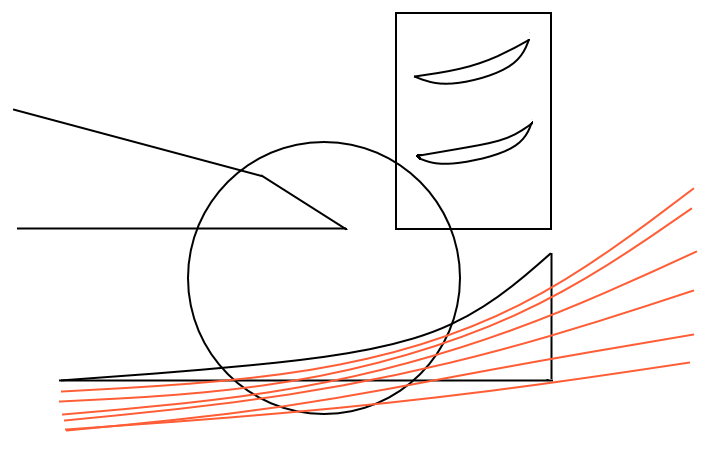The reality, as often happens, is much more complicated than what we described, but we can safely assume that increasing air speed’s with respect to the car in the area close to the ground is a diffuser’s main target. This happens in accordance to Bernoulli’s theorem.

Diffusers are extremely important elements in a racecar aerodynamics because, as the picture at the opening of this article shows, they produce downforce in a very “efficient” way, this meaning (as we will see in the next article) that they produce downforce at the cost of a relatively small amount of drag.

Diffusers are extremely complex devices and work much more tridimensionally than what our simple explanation tells. Since the sides of the diffuser are at a distance from the ground and don’t seal the volume below the car (as it actually happened at the beginning of “ground effect era” in Formula 1), the low pressure zone the diffuser creates soaks air also from the sides of the floor, creating vortices that plays a very important role on downforce generation. The following picture, taken from Katz book “Race Car Aerodynamics: Designing for Speed”, shows what we are talking about.To partially drive and control these vortexes, diffusers often present vertical walls that divide their volume into smaller sub-compartments.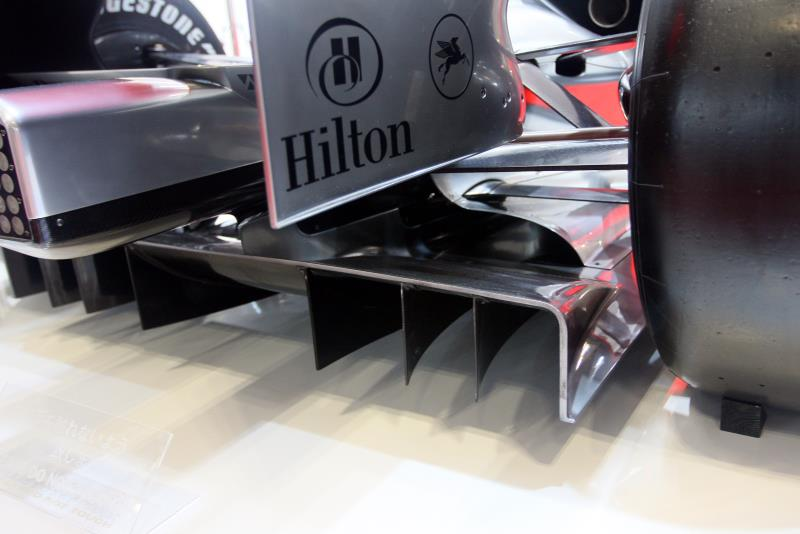Since they basically create a lower pressure area below the car that “sucks” air from under the floor, diffuser’s effectiveness increases when a wing is positioned close to their exit because the wing creates a further lower pressure area on its lower side, that increases the extraction power of the whole diffuser-wing system.Diffuser’s ability to extract air from under the car and, consequently, to create downforce, is closely linked to how close they are to the ground and, hence, to car’s ride heights. As we will see more in details in one of the following articles, this is one of the reasons why controlling ride heights is so critical in high downforce cars, like LMP vehicles.